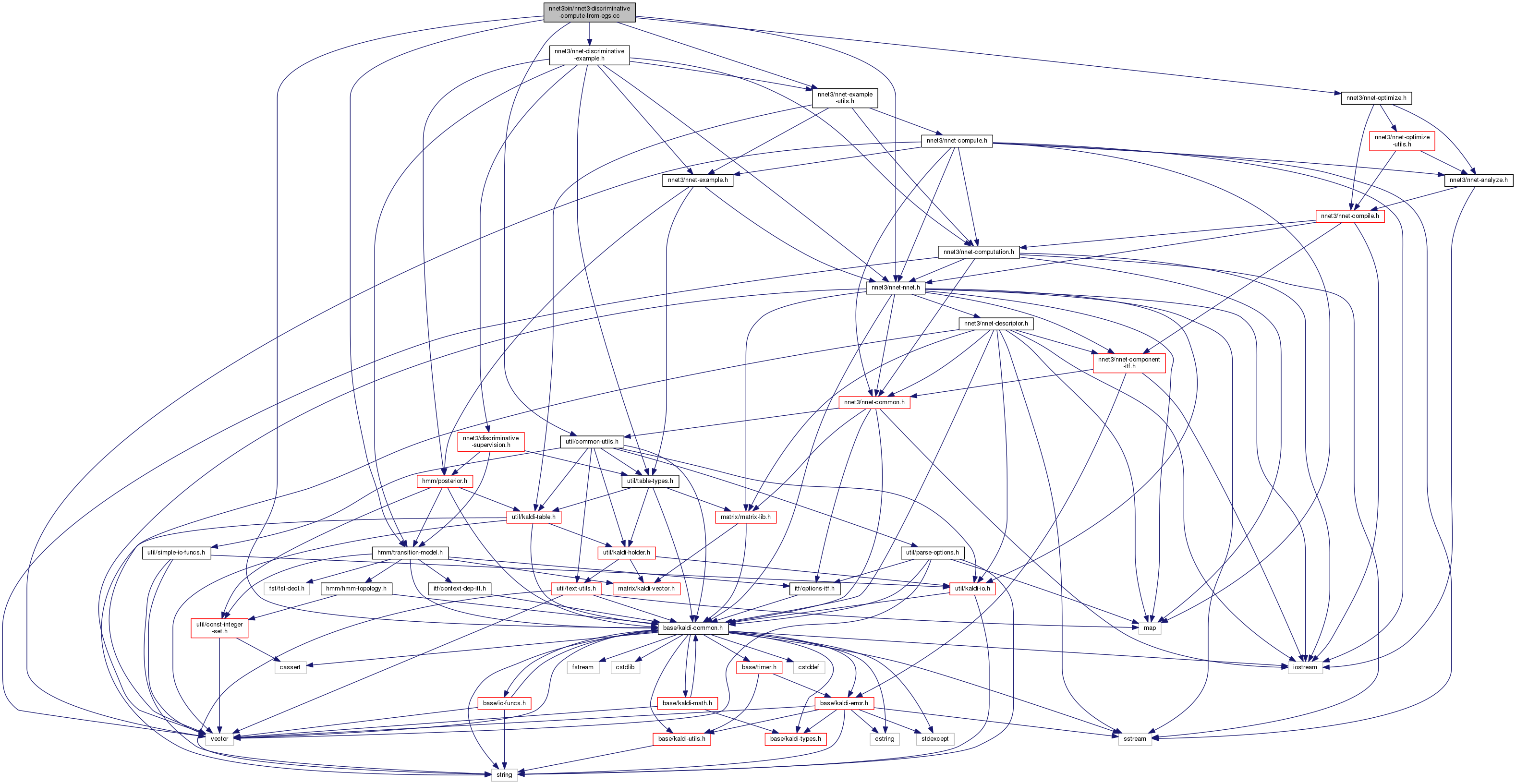nnet3-discriminative-compute-from-egs.cc File Reference
`#include "base/kaldi-common.h"`
`#include "util/common-utils.h"`
`#include "hmm/transition-model.h"`
`#include "nnet3/nnet-nnet.h"`
`#include "nnet3/nnet-example-utils.h"`
`#include "nnet3/nnet-discriminative-example.h"`
`#include "nnet3/nnet-optimize.h"`
Include dependency graph for nnet3-discriminative-compute-from-egs.cc:Go to the source code of this file.

## Classes

class  NnetComputerFromEg

## Namespaces

kaldi
This code computes Goodness of Pronunciation (GOP) and extracts phone-level pronunciation feature for mispronunciations detection tasks, the reference:

kaldi::nnet3

## Functions

int main (int argc, char *argv[])

## ◆ main()

 int main ( int argc, char * argv[] )

Definition at line 63 of file nnet3-discriminative-compute-from-egs.cc.

63  {
64  try {
65  using namespace kaldi;
66  using namespace kaldi::nnet3;
67  typedef kaldi::int32 int32;
68  typedef kaldi::int64 int64;
69
70  const char *usage =
71  "Read input nnet discriminative training examples, and compute the "
72  "output for each one. This program is similar to "
73  "nnet3-compute-from-egs, but works with discriminative egs. \n"
74  "If --apply-exp=true, apply the Exp() function to the output before writing\n"
75  "it out.\n"
76  "Note: This program uses only the input; it does not do forward-backward\n"
77  "over the lattice. See nnet3-discriminative-compute-objf for that.\n"
78  "\n"
79  "Usage: nnet3-discriminative-compute-from-egs [options] <raw-nnet-in> <training-examples-in> <matrices-out>\n"
80  "e.g.:\n"
81  "nnet3-discriminative-compute-from-egs --apply-exp=true 0.raw ark:1.degs ark:- | matrix-sum-rows ark:- ... \n"
83
84  bool binary_write = true,
85  apply_exp = false;
86  std::string use_gpu = "yes";
87
88  ParseOptions po(usage);
89  po.Register("binary", &binary_write, "Write output in binary mode");
90  po.Register("apply-exp", &apply_exp, "If true, apply exp function to "
91  "output");
92  po.Register("use-gpu", &use_gpu,
93  "yes|no|optional|wait, only has effect if compiled with CUDA");
94
96
97  if (po.NumArgs() != 3) {
98  po.PrintUsage();
99  exit(1);
100  }
101
102 #if HAVE_CUDA==1
103  CuDevice::Instantiate().SelectGpuId(use_gpu);
104 #endif
105
106  std::string nnet_rxfilename = po.GetArg(1),
107  examples_rspecifier = po.GetArg(2),
108  matrix_wspecifier = po.GetArg(3);
109
110  Nnet nnet;
112
113  NnetComputerFromEg computer(nnet);
114
115  int64 num_egs = 0;
116
118  BaseFloatMatrixWriter matrix_writer(matrix_wspecifier);
119
120  for (; !example_reader.Done(); example_reader.Next(), num_egs++) {
121  Matrix<BaseFloat> output;
122  NnetExample eg;
123  NnetDiscriminativeExample disc_eg = example_reader.Value();
124  eg.io.swap(disc_eg.inputs);
125
126  for (int32 i = 0; i < disc_eg.outputs.size(); i++) {
127  NnetIo io;
128  io.name = disc_eg.outputs[i].name;
129  io.indexes = disc_eg.outputs[i].indexes;
130  eg.io.push_back(io);
131  }
132
133  computer.Compute(eg, &output);
134  KALDI_ASSERT(output.NumRows() != 0);
135  if (apply_exp)
136  output.ApplyExp();
138  }
139 #if HAVE_CUDA==1
140  CuDevice::Instantiate().PrintProfile();
141 #endif
142  KALDI_LOG << "Processed " << num_egs << " examples.";
143  return 0;
144  } catch(const std::exception &e) {
145  std::cerr << e.what() << '\n';
146  return -1;
147  }
148 }
NnetExample is the input data and corresponding label (or labels) for one or more frames of input...
Definition: nnet-example.h:111
This code computes Goodness of Pronunciation (GOP) and extracts phone-level pronunciation feature for...
Definition: chain.dox:20
A templated class for writing objects to an archive or script file; see The Table concept...
Definition: kaldi-table.h:368
kaldi::int32 int32
std::vector< Index > indexes
"indexes" is a vector the same length as features.NumRows(), explaining the meaning of each row of th...
Definition: nnet-example.h:42
void ReadKaldiObject(const std::string &filename, Matrix< float > *m)
Definition: kaldi-io.cc:832
The class ParseOptions is for parsing command-line options; see Parsing command-line options for more...
Definition: parse-options.h:36
A templated class for reading objects sequentially from an archive or script file; see The Table conc...
Definition: kaldi-table.h:287
#define KALDI_ASSERT(cond)
Definition: kaldi-error.h:185
MatrixIndexT NumRows() const
Returns number of rows (or zero for empty matrix).
Definition: kaldi-matrix.h:64
std::vector< NnetIo > inputs
&#39;inputs&#39; contains the input to the network– normally just it has just one element called "input"...
std::vector< NnetDiscriminativeSupervision > outputs
&#39;outputs&#39; contains the sequence output supervision.
std::string name
the name of the input in the neural net; in simple setups it will just be "input".
Definition: nnet-example.h:36
std::vector< NnetIo > io
"io" contains the input and output.
Definition: nnet-example.h:116
#define KALDI_LOG
Definition: kaldi-error.h:153
NnetDiscriminativeExample is like NnetExample, but specialized for sequence training.